Math Concepts

# Know the "What, Where and How" of Histograms

0

## Table of Contents

 1 Introduction 2 When to use histograms? 3 Types of Histograms based on the types of data 4 Best practices for using Histogram 5 Applications in Real Life 6 Common misuses 7 Summary 8 Frequently Asked Questions (FAQs) 9 External References

19 November  2020

Reading Time: 5 Minutes

## Introduction

As we all know, a graph refers to a diagram which shows us the relationship between two or more things. Therefore a histogram is an example of a graph.

It was first introduced by Karl Pearson, an English Mathematician and Biostatistician in 1891.

The term “histogram” is derived from two words: historical diagram, which is the histogram’s function, to display past data.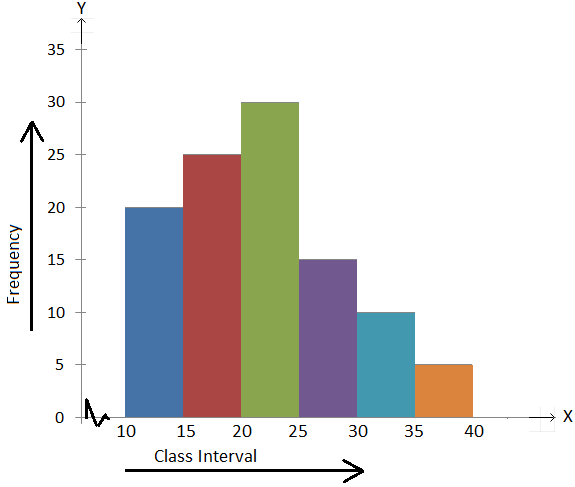A histogram is a graphical representation that organizes a group of data points into user-specified ranges.

### Difference between histogram and bar graph

It is very similar to a bar graph, but a histogram groups numbers into ranges or bins.

Also, a histogram, unlike a vertical bar graph, shows no gaps between the bars. The height of each bar shows how many fall into each range.

Also read:

The histogram condenses a series of data into an easily interpreted visual by taking many data points and grouping them into logical ranges or bins.

A histogram is a representation of data that buckets a range of outcomes into columns along the x-axis.  The y-axis represents the number count or percentage of occurrences in the data for each column and can be used to visualize data distributions.

A histogram is the most commonly used graph to show frequency distributions. A frequency distribution shows how often each different value in a set of data occurs.

This helpful data collection and analysis tool is considered one of the seven basic quality tools.

## Know the "What, Where and How" of Histograms

A histogram is a graphical display of data with bars of different heights, where each bar groups numbers into ranges. The taller the bars, the more the data falls in that range. It displays the shape as well as the spread of continuous sample data.

Know the "What, Where and How" of Histograms is mentioned below in the Downloadable PDF.

 📥 Know the "What, Where and How" of Histograms Download

## When to use histograms?

When you have a large set of measurements presented in a table, a Histogram can organize and display the data in a more user-friendly manner. A Histogram makes it easier to see where the majority of values fall in a measurement scale and how much variation there is.

Histograms are useful for showing general distributional features of dataset variables. You can see roughly where the distribution peaks are, whether the distribution is skewed or symmetric.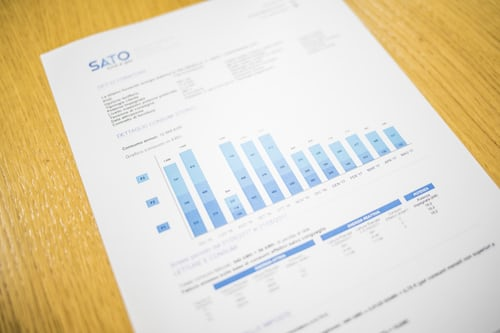They are used when large data sets need to be summarized graphically and compare results with specification limits. This helps in identifying the values that frequently occur quickly.

Histograms are most handy to use and interpret data when the data is numerical; if you want to see the shape of the data’s distribution, especially when determining whether a process’s output is distributed approximately normally.

Also helpful to check whether a process change has occurred from one time period to another. Compared to other summarizing methods, histograms have the most prosperous descriptive power while being the fastest way to interpret data – the human brain prefers visual perception.

## Types of Histograms based on the types of data

### A normal distribution

In a normal distribution, points on both sides of the average are alike.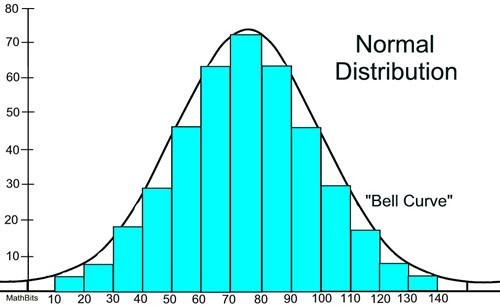### A bimodal distribution

In a bimodal distribution, there are two peaks. The data is separately analyzed as a normal distribution. Therefore they are represented as two different peaks.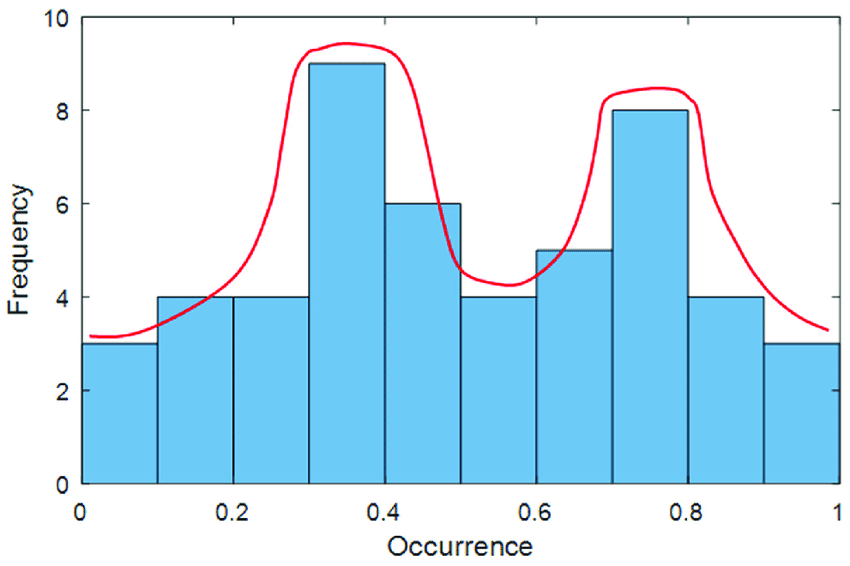### A right-skewed or positive skewed distribution

A right-skewed distribution is also called a positively skewed distribution.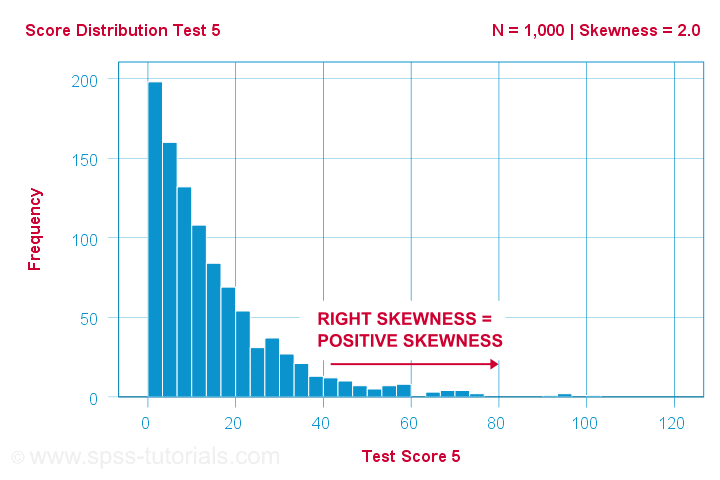In a right-skewed distribution, a large number of data values occur on the left side with a fewer number of data values on the right side. A right-skewed distribution usually occurs when the data on the left-hand side of the histogram has a low range boundary; for example, 0.

### A left-skewed or negatively skewed distribution

A left-skewed distribution is also called a negatively skewed distribution.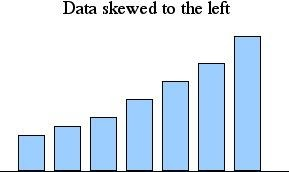In a left-skewed distribution, a large number of data values occur on the right side with a fewer number of data values on the left side.

A random distribution:

There is no pattern in a random distribution. Therefore it has several peaks and the data should be separated and analyzed separately. The reason behind this could be that the data properties were combined.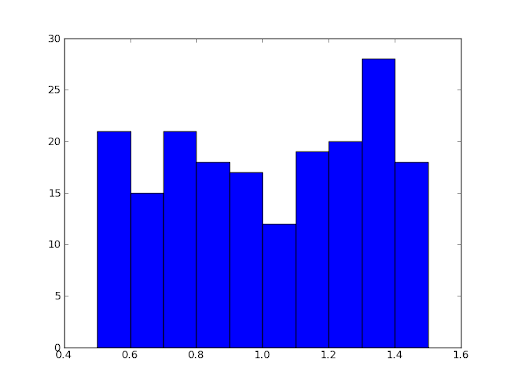## Best practices for using Histogram

### Use a zero-valued baseline

An important aspect of histograms is that they must be plotted with a zero-valued baseline.

Since each bar’s height implies the frequency of data in each bin, changing the baseline, or introducing a gap in the scale will skew the perception of the distribution of data.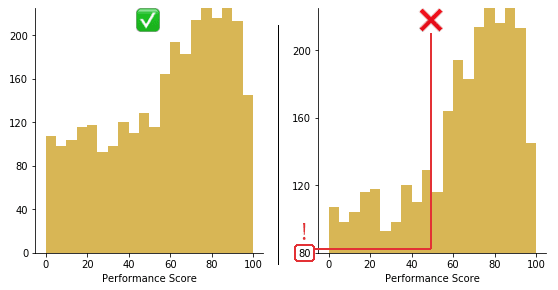### Bin Carefully

Histograms are column-charts, in which each column represents a range of the values, and the height of a column corresponds to how many values are in that range.

The wider the range (bin width), the fewer columns (bins) there will be.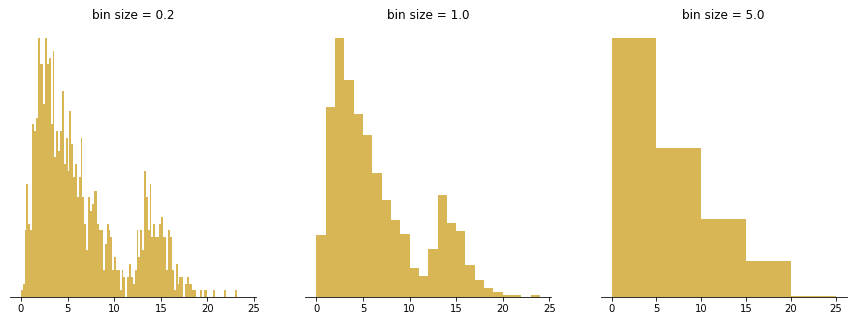Bins that are too wide can hide important details about the distribution while bins that are too narrow can cause a lot of noise and hide important information about the distribution as well.

The width of the bins should be equal, and you should only use round values like 1, 2, 5, 10, 20, 25, 50, 100, and so on to make it easier for the viewer to interpret the data.

### Choose interpretable bin boundaries

Labels typically should fall on the bin boundaries to best inform where the limits of each bar lie. Labels don’t need to be set for every bar, but having them between every few bars helps the reader keep track of value.

Top: Carelessly splitting the data into ten bins from min to max can end up with some very odd bin divisions.

Bottom: fewer tick marks are needed when the bin size is easy to follow.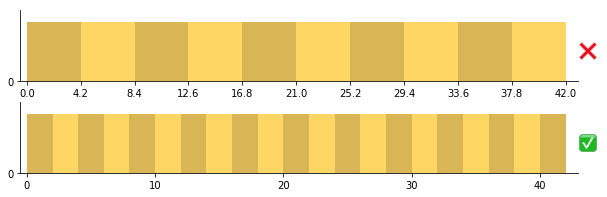## Applications of Histogram in Real Life

A histogram can be used in numerous places and situations in real life. Some of the commonly used fields are as follows:• Stock Exchanges: Histograms are used to identify trade at different places or different groups of investors.
• Medical and Clinical research: Histograms can be used to identify the presence or absence of a condition among different categories of people.
• Photography: Histograms are used for image processing and digitization.
• Six Sigma: Histograms are used to study defect patterns across different categories of samples.

## What are the common misuses of histograms?

### Measured variable is not continuous numeric

As noted in the opening sections, a histogram is meant to depict the frequency distribution of a continuous numeric variable.

When our variable of interest does not fit this property, we need to use a different chart type instead: a bar chart. However, there are certain variable types that can be trickier to classify: those that take on discrete numeric values and those that take on time-based values.

Variables that take discrete numeric values (e.g. integers 1, 2, 3, etc.) can be plotted with either a bar chart or histogram, depending on the context.

Using a histogram will be more likely when there are a lot of different values to plot. When the range of numeric values is large, the fact that values are discrete tends to not be important and continuous grouping will be a good idea.A trickier case is when the variable is a time-based feature. When values correspond to relative periods of time (e.g. 30 seconds, 20 minutes), then binning by time periods for a histogram makes sense.

However, when values correspond to absolute times (e.g. January 10, 12:15) the distinction becomes blurry. When new data points are recorded, values will usually go into newly-created bins, rather than within an existing range of bins.

In addition, certain natural grouping choices, like by month or quarter, introduce slightly unequal bin sizes. For these reasons, it is better to use a different chart type like a bar chart or line chart.

### Using unequal bin sizes

Creating a histogram with bins of unequal size is not strictly a mistake, but one has to be careful in how the histogram is created as it can cause a lot of difficulties in interpretation.

When bin sizes are consistent, this makes measuring bar area and height equivalent. In a histogram with variable bin sizes, however, the height can no longer correspond with the total frequency of occurrences. Doing so would distort the perception of how many points are in each bin since increasing a bin’s size will only make it look bigger.

## Summary

A histogram tool is a standard tool for understanding data and the characteristics of data.

Knowing how to correctly read a histogram graph can greatly assist in process improvement efforts.

A histogram is a representation of data that buckets a range of outcomes into columns along the x-axis.  The y-axis represents the number count or percentage of occurrences in the data for each column and can be used to visualize data distributions.

A histogram is the most commonly used graph to show frequency distributions. A frequency distribution shows how often each different value in a set of data occurs.

This helpful data collection and analysis tool is considered one of the seven basic quality tools.

A histogram's common use also makes an excellent graphic for representing data during presentations.

Why don't you try developing a histogram yourself?

## About Cuemath

Cuemath, a student-friendly mathematics platform, conducts regular Online Live Classes for academics and skill-development and their Mental Math App, on both iOS and Android, is a one-stop solution for kids to develop multiple skills. Know more about the Cuemath fee here, Cuemath Fee

## What is data?

Data is a collection of facts, such as numbers, words, measurements, observations, or just descriptions of things.

## How do you differentiate between data and information?

Data is unorganized, while information is structured or organized. Data is a collection of facts. Information is how you understand those facts in context.

## What are the ways to represent data?

Tables, charts, and graphs are all ways of representing data, and they can be used for two general purposes. The first is to support the collection, organization, and analysis of data as part of a scientific study. The second is to help present the conclusions of a survey to a broader audience.

## What are the different types of graphs?

The four most common are line graphs, bar graphs and histograms, pie charts, and Cartesian graphs.

## What is the difference between histogram and bar graph?

With bar charts, each column represents a group defined by a categorical variable; and with histograms, each column represents a group defined by a continuous, quantitative variable.

It is very similar to a bar graph, but a histogram groups numbers into ranges or bins.

Also, a histogram, unlike a vertical bar graph, shows no gaps between the bars. The height of each bar shows how many fall into each range.

## External References

To learn more about histograms, check these out:

Related Articles
GIVE YOUR CHILD THE CUEMATH EDGE
Access Personalised Math learning through interactive worksheets, gamified concepts and grade-wise courses
Learn More About Cuemath